Courses

# RD Sharma Solutions -Page No.9.19, Ratio Proportion And Unitary Method, Class 6, Maths Class 6 Notes | EduRev

## Class 6 : RD Sharma Solutions -Page No.9.19, Ratio Proportion And Unitary Method, Class 6, Maths Class 6 Notes | EduRev

The document RD Sharma Solutions -Page No.9.19, Ratio Proportion And Unitary Method, Class 6, Maths Class 6 Notes | EduRev is a part of the Class 6 Course RD Sharma Solutions for Class 6 Mathematics.
All you need of Class 6 at this link: Class 6

Question 1: A ratio equivalent of 2 : 3 is

(a) 4 : 3
(b) 2 : 6
(c) 6 : 9
(d) 10 : 9

2 : 3 is equivalent to 6 : 9. (Dividing by 3)

Question 2: The angles of a triangle are in the ratio 1 : 2 : 3. The measure of the largest angle is
(a) 30°
(b) 60°
(c) 90°
(d) 120°

Sum of all the angles of a triangle = 180o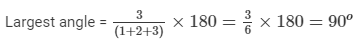Question 3: The sides of a triangle are in the ratio 2 : 3 : 5. If its perimeter is 100 cm, the length of its smallest side is
(a) 2 cm
(b) 20 cm
(c) 3 cm
(d) 5 cm

Length of the smallest side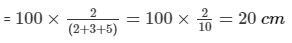Disclaimer: In actual terms, the sides of a triangle cannot be in the ratio 2 : 3 : 5.
Because, to form a triangle, the sum of two sides must be greater than the third side.
Here, the sum of two sides of the triangle is equal to the third side.

Question 4: Two numbers are in the ratio 7 : 9. If the sum of the numbers is 112, then the larger number is

(a) 63
(b) 42
(c) 49
(d) 72

Let the larger number be x.
Then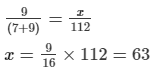Question 5: Two ratio 384 : 480 in its simplest form is
(a) 3 : 5
(b) 5 : 4
(c) 4 : 5
(d) 2 : 5

384/480 = 4/5 (Dividing the denominator and numerator by 96)

Question 6: If A, B, C,divide Rs 1200 in the ratio 2 : 3 : 5, then B's share is
(a) Rs 240
(b) Rs 600
(c) Rs 380
(d) Rs 360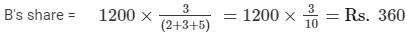Question 7: If a bus travels 126 km in 3 hours and a train travels 315 km in 5 hours, then the ratio of their speeds is
(a) 2 : 5
(b) 2 : 3
(c) 5 : 2
(d) 25 : 6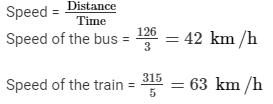Ratio of their speeds = 42 : 63 = 2 : 3

Question 8: The ratio of male and female employees in a multinational company is 5 : 3. If there are 115 male employees in the company, then the number off female employees is

(a) 96
(b) 52
(c) 69
(d) 66

If the number of male employees is 115, let the number of female employees be x.

According to the question: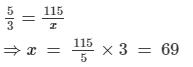Question 9: Length and width of a field are in the ratio 5 : 3. If the width of the field is 42 m, then its length is

(a) 50 m

(b) 70 m

(c) 80 m

(d) 100 m

Ratio of length and width of the field = 5 : 3

Let the length be x m.

∵ Width = 42 m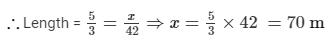Question 10: If 57 : x = 51 : 85, then the value of x is

(a) 95
(b) 76
(c) 114
(d) None of these

Consider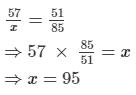Question 12: If 4, a, a, 36 are in proportion, then a =
(a) 24
(b) 12
(c) 3
(d) 24
(b) 12

4, a, a, 36 are in proportion; therefore, we get:

4 : a : : a : 36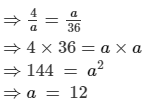#### Question 13: If 5 : 4 : : 30 : x, then the value of x is(a) 24(b) 12(c) 3/2(d) 6 ANSWER: (a) 245 : 4 : : 30 : x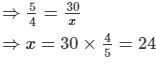Question 14: If a, b, c, d are in proportion, then

(a) ab = cd

(b) ac = bd

(d) None of these

∵ a, b, c and d are in proportion.

∴ a : b = c : d

⇒a/b = c/d

Question 15: The weight of 45 folding chairs is 18 kg.How many such chairs can be loaded on a truck having a capacity of carrying 4000 kg load?

ANSWER: Number of folding chairs weighing 18 kg = 45

Number of folding chairs weighing 1 kg = 45/18

Number of folding chairs weighing 4,000 kg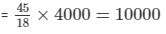103 docs

,

,

,

,

,

,

,

,

,

,

,

,

,

,

,

,

,

,

,

,

,

,

,

,

,

,

,

,

,

,

;